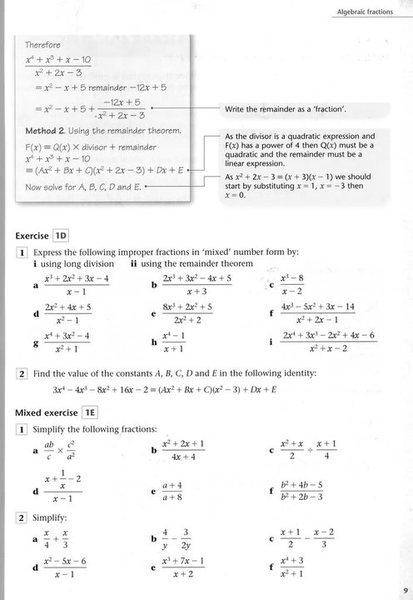# Polynominal and the remainder theorom

## Homework Statement

Using the remainder theorem solve

$$\frac{8x^{3} + 2x^{2} + 5}{2x^{2}+2}$$

## Homework Equations

The remainder theorem:

$$F(x) \equiv Q(x) \times divisor + remainder$$

## The Attempt at a Solution

$$8x^{3} + 2x^{2} + 5 \equiv (Ax+B)(2x^{2}+2) + C$$

I can do it for linear divisors but i dont think i've set this out correctly for a quadratic divisor
Any suggestions. Thanks

Last edited:

morphism
Homework Helper
Solve what exactly?

The remainer term should be Cx + D since the divisor is quadratic. Multiply everything on the right side out and compare them to the coefficients of the left hand side. For example, the only cubic term on the right will be Ax^3 so A must equal 8.

Last edited:
tiny-tim
Homework Helper
Hi thomas49th!So far as I know, the remainder theorem only applies to linear divisors.

The remainder for a quadratic divisor will not generally be a number.As morphism says, what exactly are you trying to solve?Hi thomas49th!So far as I know, the remainder theorem only applies to linear divisors.

The remainder for a quadratic divisor will not generally be a number.As morphism says, what exactly are you trying to solve?He said remainder theorem, but his statement is just polynomial division.

f(x) = q(x)g(x) + r(x)
The remainder theorem is really just a corollary to division by letting g(x) = x-a to solve for f(a).

Correction to my earlier post: The cubic on the RHS is 2Ax^3 = 8 so A =4Question Exercise 1D question 1 (e)

not sure why the remainder must be linear Cx + D. Same, with the example in the box at the top righ tof the page. What are the rules??

Thanks. Tom

tiny-tim
Homework Helper
not sure why the remainder must be linear Cx + D. Same, with the example in the box at the top righ tof the page. What are the rules??

Hi Tom!The rule is pretty much the same as for remainders in ordinary arithmetic …

The remainder must be smaller than the divisor!

So if you're dividing by 17 in ordinary arithmetic, the remainder can't be higher than 16.

And if you're dividing by x² + ax + b in polynomial fractions, the remainder must be less than x², so it must be linear.

By coincidence, in the case you're trying to solve, the Q(x) must be linear also, because the largest power in Q(x) will always be the largest power in the divided minus the largest power in the divisor … in this case, 3 minus 2

remainder theorem

Now I see where the remainder theorem comes in.

To use the letters in the book, you need to find A B C D and E.

And you can find D and E quickly by using the remainder theorem on each linear factor, one at a time.

In this case, if you haven't done complex numbers yet, then 2x² + 2 has no factors, and so you can't use the remainder theorem.

But if you have done complex numbers, then you can get D and E from Di + E and -Di + E, which you get from substituting x = i and x = -i.There's no need for complex numbers here. You guys are confusing two related theorems because of the name that book uses. The remainder theorem says that if f(x) = q(x)*(x-a) + r(x) then f(a) = r(a). Notice the linear term is needed since it goes to zero when x = a. The question here has nothing to do with that theorem, but with polynomial division.

8x^3 + 2x^2 + 5 = (Ax+B)*(2x^2+2) + Cx + D.
(Ax+B) must be linear since the highest power on the left is a cubic, and the remainder must be linear since otherwise 2x^2+2 would still divide into it.
Expand out the right hand side.
8x^3 + 2x^2 + 5 = Ax^3 + 2Bx^2 + 2Ax + 2B + Cx + D
Regroup all the terms with similar powers of x.
8x^3 + 2x^2 + 5 = 2Ax^3 + 2Bx^2 + (2A+C)x + D + 2B
Then set all the coefficients on right equal to the corresponding coefficients on the left.
2A = 8
2B = 2
2A+C = 0
D + 2B= 5
You can solve this from here, right?

Notice that we get a linear term so we could use the remainder theorem if we wanted to evaluate 8^3+2x^2+5 at x = -1/4

Last edited:
tiny-tim
And since it's his book's method, he does need to know how to do it.